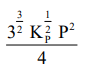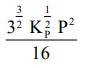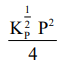Deepak Scored 45->99%ile with Bounce Back Crack Course. You can do it too!

# Consider the reaction,

Question:

Consider the reaction,

$\mathrm{N}_{2}(\mathrm{~g})+3 \mathrm{H}_{2}(\mathrm{~g}) \rightleftharpoons 2 \mathrm{NH}_{3}(\mathrm{~g})$

The equilibrium constant of the above reaction is $\mathrm{K}_{\mathrm{P}}$. If pure ammonia is left to dissociate, the partial pressure of ammonia at equilibrium is given by

(Assume that $\mathrm{P}_{\mathrm{NH}_{3}}<<\mathrm{P}_{\text {total }}$ at equilibrium)

1.2.3.4.Correct Option: , 2

Solution: